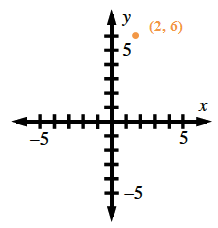### Home > CCA > Chapter 11 > Lesson 11.2.1 > Problem11-39

11-39.

Given the quadratic function $f(x)=−2(x−2)^2+6$

1. Without drawing a graph, tell the coordinates of the vertex and tell if the vertex represents the maximum or minimum value of the function.

The graphing form of a quadratic is $f(x)=a(x−h)+k$ where the vertex is at the point $(h,k)$.
If the vertex is the highest point on the graph, then it is a maximum.
If it is the lowest point on the graph, then it is a minimum.